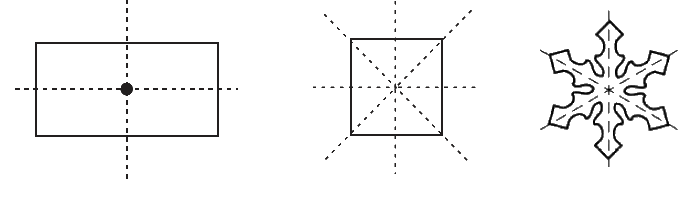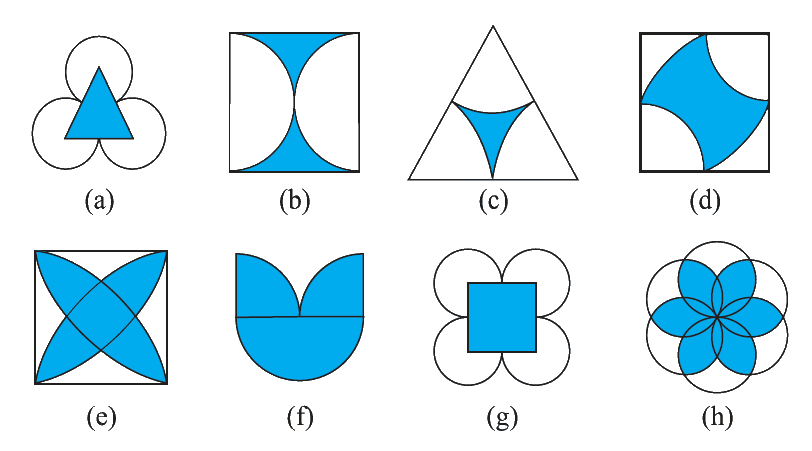# Ex.14.1 Q4 Symmetry - NCERT Maths Class 7

Go back to  'Ex.14.1'

## Question

The following figures have more than one line of symmetry. Such figures are said to have multiple lines of symmetry.Identify multiple lines of symmetry, if any, in each of the following figures:Video Solution
Symmetry
Ex 14.1 | Question 4

## Text Solution

What is known?

Different figures.

What is unknown?

Multiple lines of symmetry.

Reasoning:

In this question you have to identify multiple of lines of symmetry. For this cut the figure by using different lines, the line which cuts the figure in two same parts are called lines of symmetry and a figure has more than one line of symmetry then we can say that it has multiple lines of symmetry.

Steps:(a) It has three lines of symmetry.

(b) It has two lines of symmetry.

(c) It has three line of symmetry.

(d) It has two line of symmetry.

(e) It has four lines of symmetry.

(f) It has one line of symmetry.

(g) It has four lines of symmetry.

(h) It has six lines of symmetry.

Learn from the best math teachers and top your exams

• Live one on one classroom and doubt clearing
• Practice worksheets in and after class for conceptual clarity
• Personalized curriculum to keep up with school